Everyday maths 2

Start this free course now. Just create an account and sign in. Enrol and complete the course for a free statement of participation or digital badge if available.

Free course

# 3 Volume

The volume of a shape is how much space it takes up. You might need to calculate the volume of a space or shape if, for example, you wanted to know how much soil to buy to fill a planting box or how much concrete you need to complete your patio.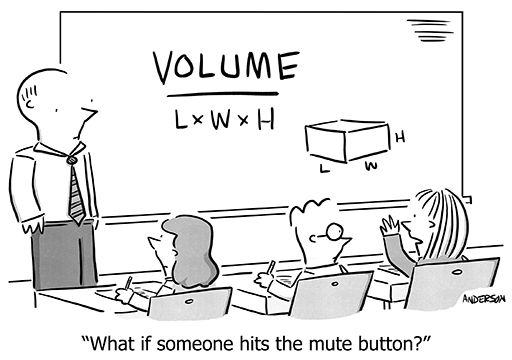Figure 33 A volume cartoon

You’ll need your area skills in order to calculate the volume of a shape. In fact, as you already know how to calculate the area of most shapes, you are one simple step away from being able to find the volume of most shapes too!

Download this video clip.Video player: bltl_4_3_3d_shapes.mp4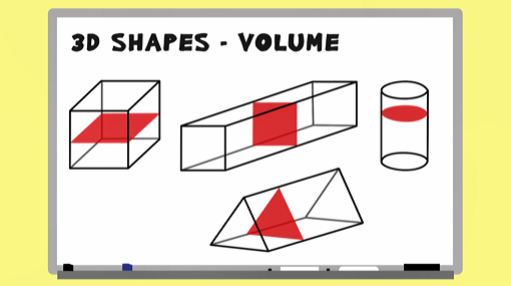Skip transcript

#### Transcript

In this video, you'll look at how to calculate the volume of prisms. A prism is a 3D shape where the cross section, or slice, of the shape is the same all the way through. You can see that a slice of any of these shapes would remain the same wherever you cut it. To calculate the volume of any prism, all you need to do is find the area of the cross section and then multiply this area by the length of the prism.

In this example, you firstly need to calculate the area of the rectangular cross section. 12 times 10 gives an area of 120 centimetres squared. Now you know the area of the cross section, all you need to do is multiply it by the length of the shape: 5 centimetres. Volume equals 120 times 5, which equals 600 centimetres cubed.

Let's try again with a different shape. Here, the cross section is the triangle. You'll recall from your earlier work that to find the area of a triangle, you use this formula. So the area of this cross section is 6 times 5, divided by 2. That is 30 divided by 2. This gives an area of 15 centimetres squared. Now, multiply the area of the cross section by the length of the shape to find the volume. 15 times 12 gives a volume of 180 centimetres cubed. Look at a couple more examples of calculating the volume of prisms, before having a go yourself.

End transcript

Interactive feature not available in single page view (see it in standard view).

## Example: Calculating volume 1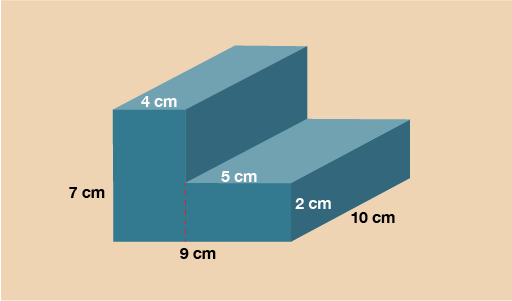Figure 34 Calculating volume 1

The cross section on this shape is the L shape on the front. In order to work out the area you’ll need to split it up into two rectangles as you practised in the previous part of this section.

Splitting vertically:

Rectangle 1 = 7 × 4 = 28 cm2

Rectangle 2 = 5 × 2 = 10 cm2

Area of cross section = 28 + 10 = 38 cm2

Now you have the area of the cross section, multiply this by the length to calculate the volume.

V = 38 × 10 = 380 cm3

## Example: Calculating volume 2

The last example to look at is a cylinder. The cross section of this shape is a circle. You’ll need to use the formula to find the area of a circle in the same way you did in the previous part of this section.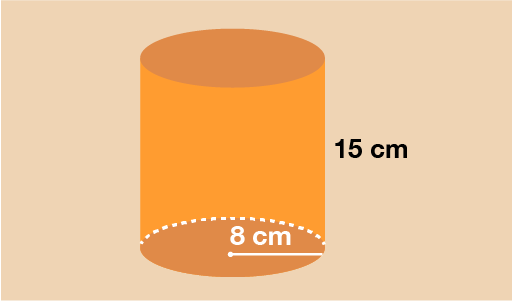Figure 35 Calculating volume 2

You can see that the circular cross section has a radius of 8 cm. To find the area of this circle, use the formula:

A = πr2

A = 3.142 × 8 × 8

A = 201.088 cm2

Now you have the area, multiply this by the length of the cylinder to calculate the volume.

V = 201.088 × 15 = 3016.32 cm3

Now try yourself in the activity below.

## Activity 7: Calculating prism volume

Work out the volume of the 3D trapezium (trapezoidal prism) shown below.

Hint: Go back to the section on area and remind yourself of the formula to find the area of a trapezium.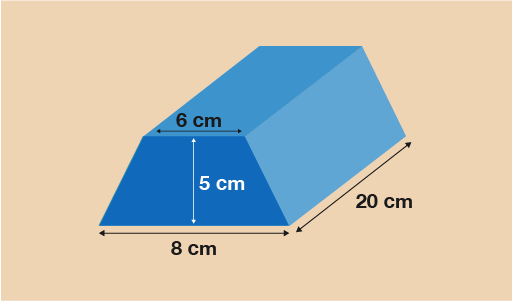Figure 36 Calculating the volume of a prism

### Answer

The cross section is a trapezium so you will need the formula:

• A =

• A =

• A =

• A =
• A = 35 cm2

Now you have the area of the cross section, multiply it by the length of the prism to calculate the volume.

• V = 35 × 20 = 700 cm3

The final part of this session will look at scale drawings and plans. It will require your previous knowledge of ratio as scale drawings are really just another application of ratio.

## Summary

In this section you have learned:

• that volume is the space inside a 3D shape or space
• how to find the volume of prisms such as cuboids, cylinders and triangular prisms.
FSM_2

### Take your learning further

Making the decision to study can be a big step, which is why you'll want a trusted University. The Open University has 50 years’ experience delivering flexible learning and 170,000 students are studying with us right now. Take a look at all Open University courses.

If you are new to University-level study, we offer two introductory routes to our qualifications. You could either choose to start with an Access module, or a module which allows you to count your previous learning towards an Open University qualification. Read our guide on Where to take your learning next for more information.

Not ready for formal University study? Then browse over 1000 free courses on OpenLearn and sign up to our newsletter to hear about new free courses as they are released.

Every year, thousands of students decide to study with The Open University. With over 120 qualifications, we’ve got the right course for you.

Request an Open University prospectus371